Diet problems

Chapter 12 Class 12 Linear Programming
Concept wise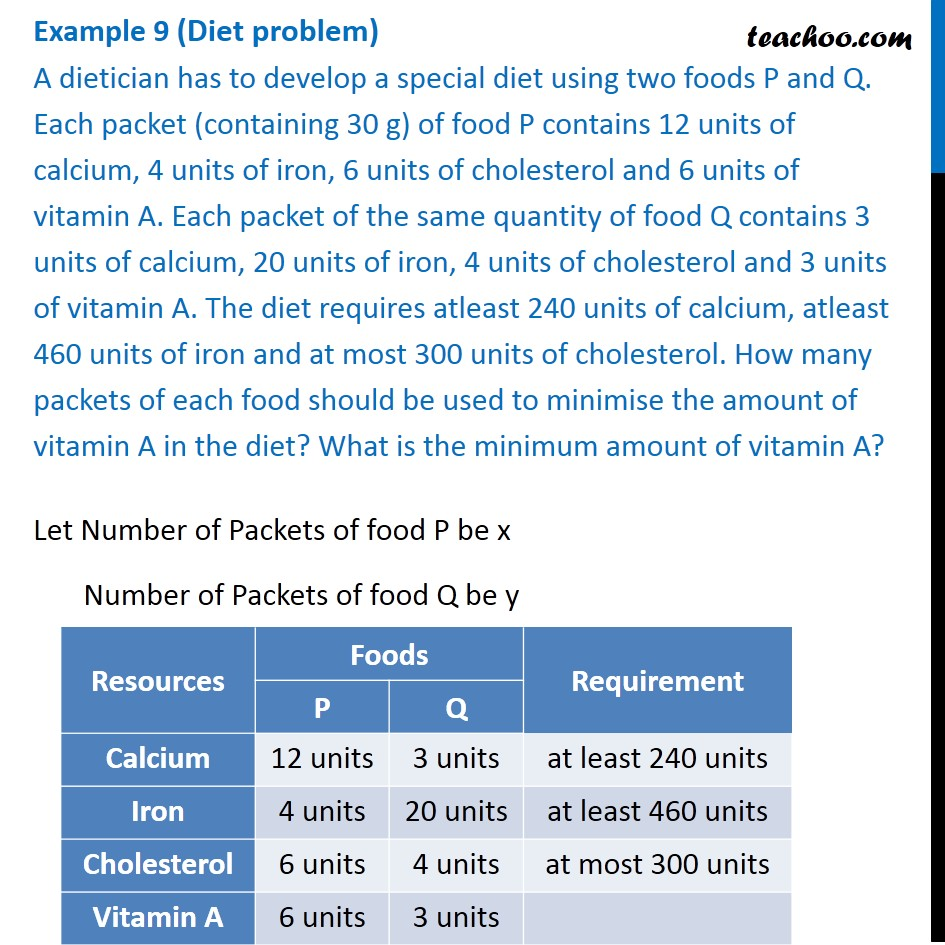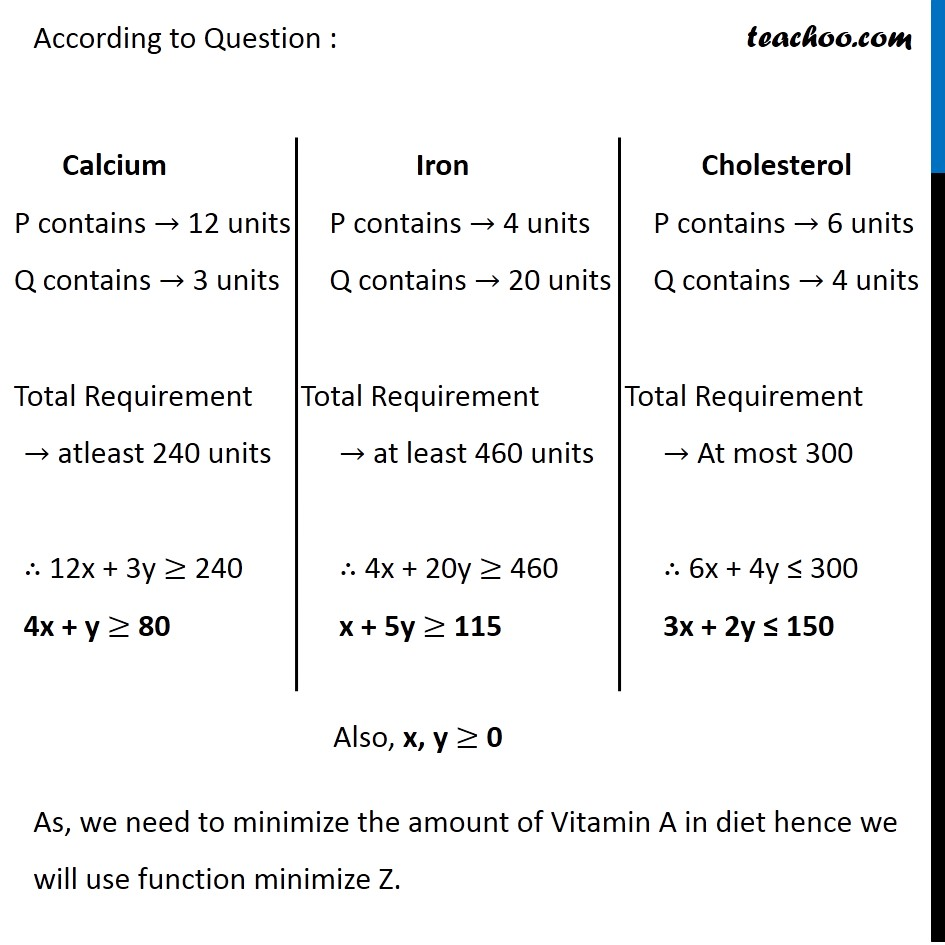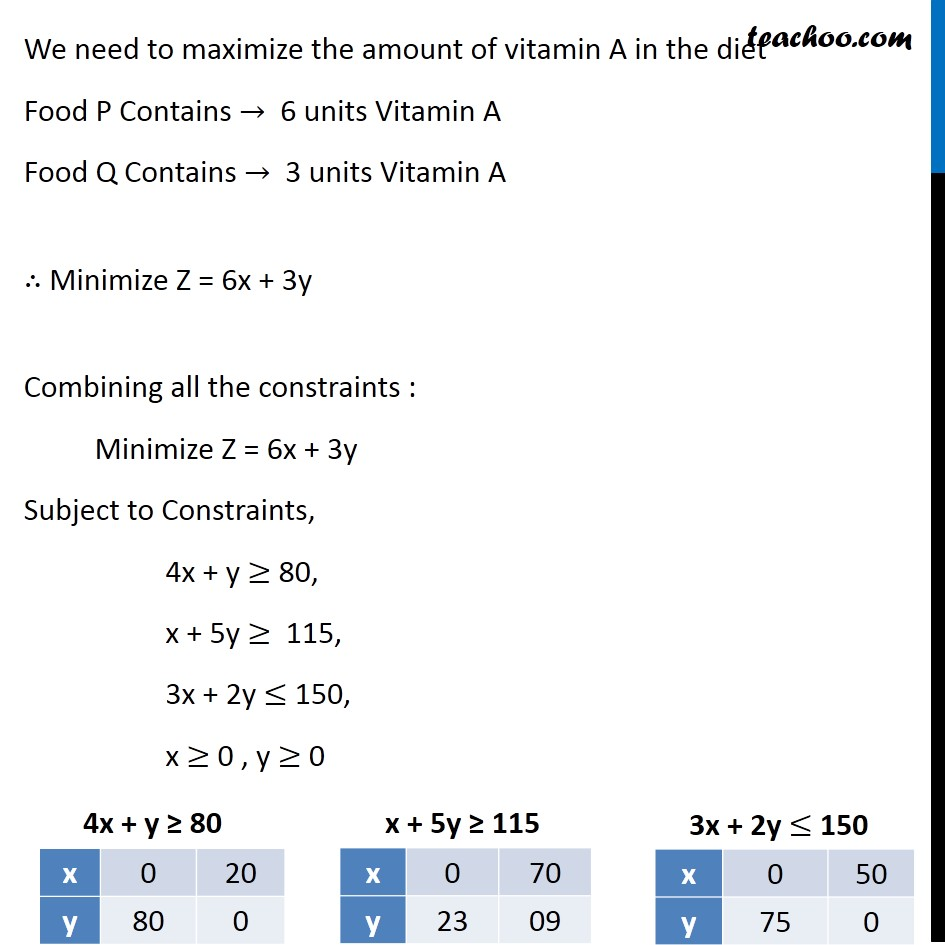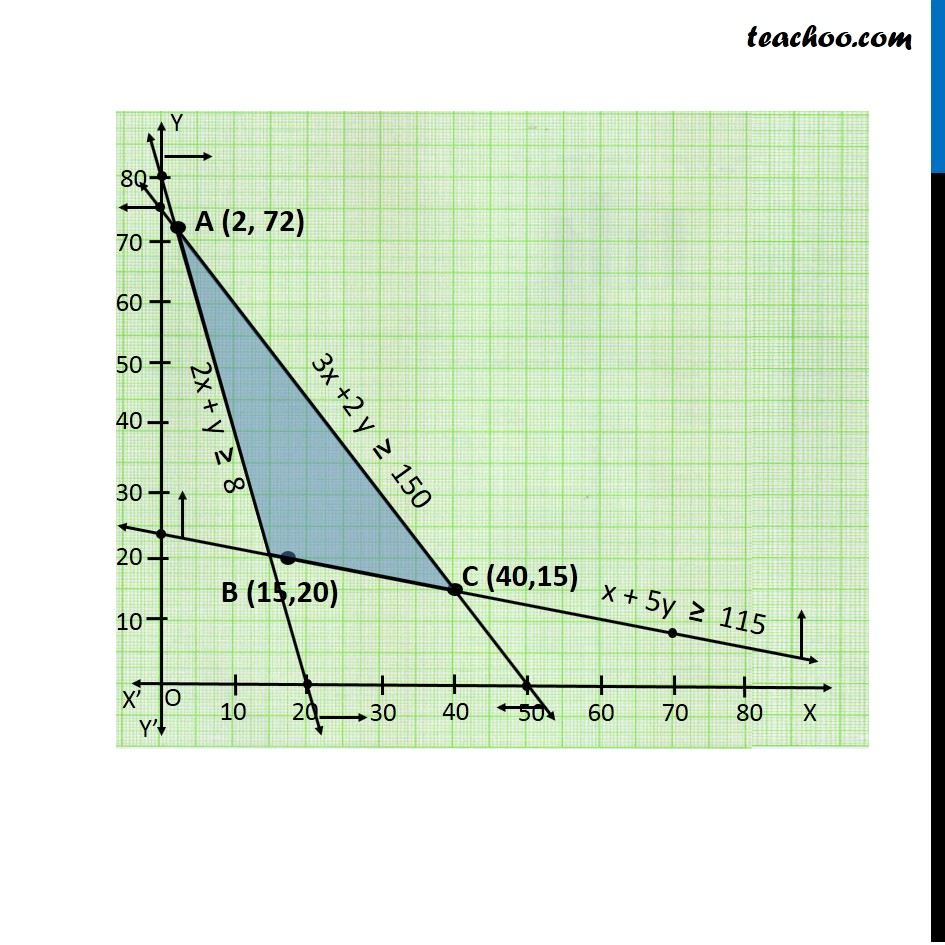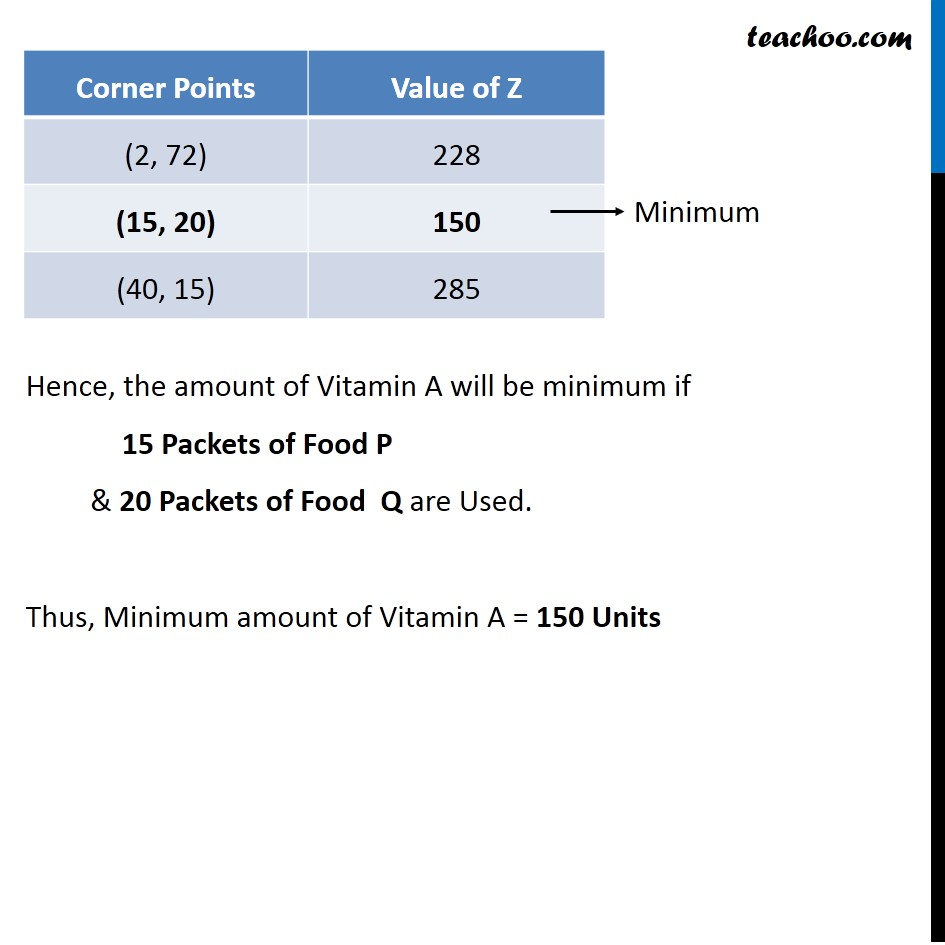Learn in your speed, with individual attention - Teachoo Maths 1-on-1 Class

### Transcript

Question 4 (Diet problem) A dietician has to develop a special diet using two foods P and Q. Each packet (containing 30 g) of food P contains 12 units of calcium, 4 units of iron, 6 units of cholesterol and 6 units of vitamin A. Each packet of the same quantity of food Q contains 3 units of calcium, 20 units of iron, 4 units of cholesterol and 3 units of vitamin A. The diet requires atleast 240 units of calcium, atleast 460 units of iron and at most 300 units of cholesterol. How many packets of each food should be used to minimise the amount of vitamin A in the diet? What is the minimum amount of vitamin A?Let Number of Packets of food P be x Number of Packets of food Q be y According to Question : As, we need to minimize the amount of Vitamin A in diet hence we will use function minimize Z. Calcium P contains → 12 units Q contains → 3 units A Total Requirement → atleast 240 units ∴ 12x + 3y ≥ 240 4x + y ≥ 80 Calcium P contains → 12 units Q contains → 3 units A Total Requirement → atleast 240 units ∴ 12x + 3y ≥ 240 4x + y ≥ 80 Iron P contains → 4 units Q contains → 20 units Total Requirement → at least 460 units ∴ 4x + 20y ≥ 460 x + 5y ≥ 115 Cholesterol P contains → 6 units Q contains → 4 units Total Requirement → At most 300 ∴ 6x + 4y ≤ 300 3x + 2y "≤" 150 We need to maximize the amount of vitamin A in the diet Food P Contains → 6 units Vitamin A Food Q Contains → 3 units Vitamin A ∴ Minimize Z = 6x + 3y Combining all the constraints : Minimize Z = 6x + 3y Subject to Constraints, 4x + y ≥ 80, x + 5y ≥ 115, 3x + 2y ≤ 150, x ≥ 0 , y ≥ 0 Hence, the amount of Vitamin A will be minimum if 15 Packets of Food P & 20 Packets of Food Q are Used. Thus, Minimum amount of Vitamin A = 150 Units